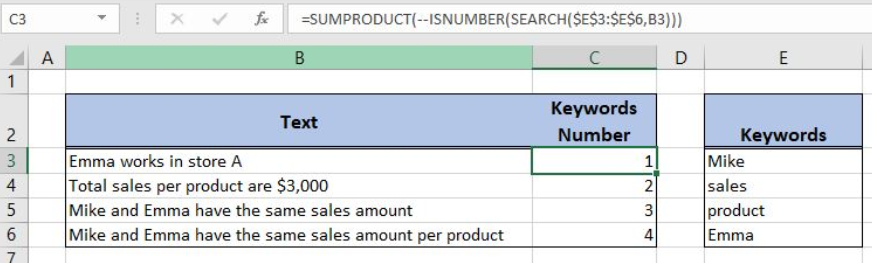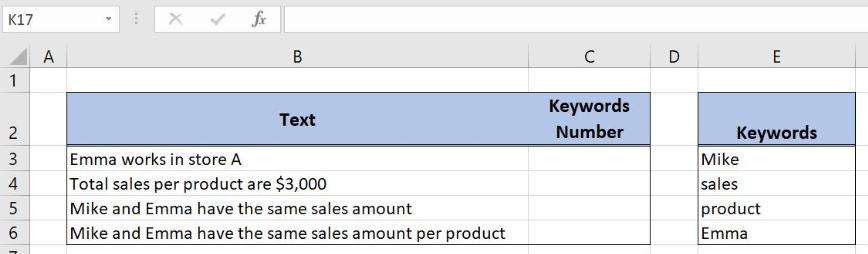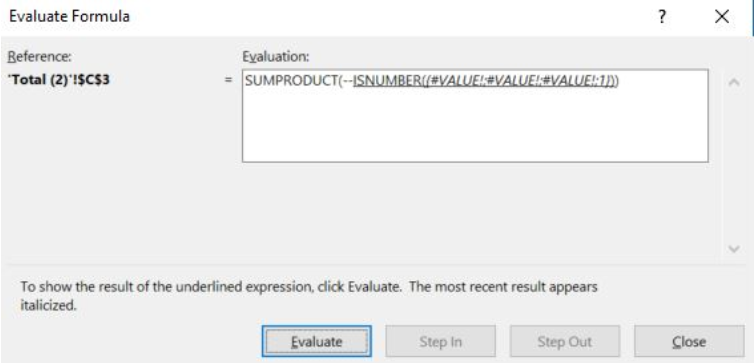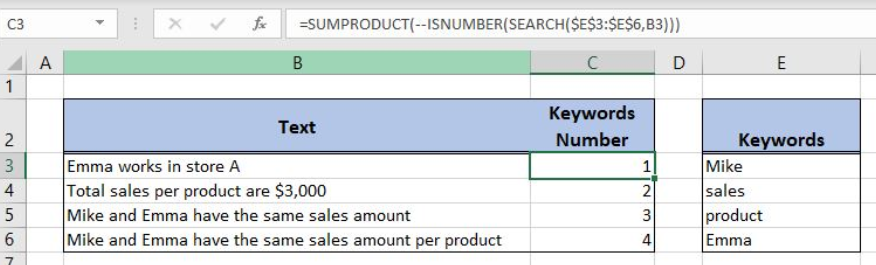Get instant live expert help with Excel or Google Sheets“My Excelchat expert helped me in less than 20 minutes, saving me what would have been 5 hours of work!”

#### Post your problem and you’ll get Expert help in seconds.

Your message must be at least 40 characters
Our professional Expert are available now. Your privacy is guaranteed.

# Count keywords cell contains

Excel allows a user to count keywords which a cell contains using the SUMPRODUCT, ISNUMBER and SEARCH functions. This step by step tutorial will assist all levels of Excel users to count keywords cell contains.Figure 1. The result of the formula

## Syntax of the SUMPRODUCT Formula

The generic formula for the SUMPRODUCT function is:

`=SUMPRODUCT(--(array))`

The parameter of the SUMPRODUCT function is:

• array – an array of values which we want to sum. The double minus before the array converts TRUE to 1 and FALSE to 0.

## Syntax of the ISNUMBER Formula

The generic formula for the ISNUMBER function is:

`=ISNUMBER(value)`

The parameter of the ISNUMBER is:

• value – a value which we want to check if it’s a number or not.

If the value is numeric, the function returns TRUE and if it’s not FALSE.

## Syntax of the SEARCH Formula

The generic formula for the SEARCH function is:

`=SEARCH(find_text, within_text, [start_num])`

The parameters of the SEARCH function are:

• find_text – a text which we want to find in another text
• within_text -a text where we want to find a find_text
• [start_num] -a position from which we want to search for a find_text. This parameter is optional. If it’s omitted, the function will search from the beginning.

If a find_text is found, the function returns the beginning position in a text. If it’s not found, the function returns an error.

## Setting up Our Data for the Formula

Let’s look at the structure of the data we will use. In column B (“Text”), we have texts where we want to find keywords. In column C (“Keyword Number”), we want to get a number of keywords in texts. In column E (“Keywords”), we have a list of keywords.Figure 2. Data that we will use in the example

## Counting Number of Keywords a Cell Contains

In our example, we want to check how many keywords from column E occurs in the cell B3 (“Emma works in store A”). The result will be in the cell C3.

The formula looks like:

`=SUMPRODUCT(--ISNUMBER(SEARCH(\$E\$3:\$E\$6, B3)))`

The parameter find_text of the SEARCH function is the \$E\$3:\$E\$6 range, while the text is B3. We must fix the find_text range, as it’s not changing when the formula is copied. The result of this function is the array, which is the value parameter of the ISNUMBER function. The result of the ISNUMBER function is the array parameter of the SUMPRODUCT function.

To apply the formula, we need to follow these steps:

• Select cell C3 and click on it
• Insert the formula: `=SUMPRODUCT(--ISNUMBER(SEARCH(\$E\$3:\$E\$6,B3)))`
• Press enter
• Drag the formula down to the other cells in the column by clicking and dragging the little “+” icon at the bottom-right of the cell.

The SEARCH function looks for keywords and returns the first position of a keyword or an error. If we evaluate the function we can see this array:Figure 3. Evaluate the formula

First three keywords are not found, and the last keyword starts from the first position, so the array is `{#VALUE!; #VALUE!; #VALUE!; 1}`.

Now the ISNUMBER converts these values to TRUE and FALSE. Therefore, the result of the ISNUMBER function is the array `{FALSE, FALSE, FALSE, TRUE}`.Figure 4. Using the formula to count keywords cell contains

Finally, the SUMPRODUCT converts TRUE and FALSE to 1 and 0, so the array is `{0, 0, 0,1}`. The sum of this array is one, which means that one of the keywords is found in the cell B3. Therefore, the result in C3 is 1.

Most of the time, the problem you will need to solve will be more complex than a simple application of a formula or function. If you want to save hours of research and frustration, try our live Excelchat service! Our Excel Experts are available 24/7 to answer any Excel question you may have. We guarantee a connection within 30 seconds and a customized solution within 20 minutes.

### Did this post not answer your question? Get a solution from connecting with the expert.Another blog reader asked this question today on Excelchat:
Solution examplesI need to count number of dates from a timestamped date in column from the completed date column to show the number of days. I have not successfully been able to do this. I am a novice Excel user. Can you help?
Solved by O. B. in 15 minsUse the Vlookup Function to complete the "employee" column of table 2. Use "job Id" from table 2 as your lookup_value(s) and table 1 as your reference.
Solved by C. H. in 16 minsIf a cell in another sheet is populated I need a vlookup done. If the cell is not populated I need the cell to return blank.
Solved by T. D. in 60 minsI am trying to make a chart that turns a week range red if nothing is entered in the range. If something is entered then I would like it to turn green. Please Help
Solved by E. U. in 43 minsI need a check box to show/hide an answer of an if function
Solved by Z. U. in 23 mins## Subscribe to Excelchat.coAnother blog reader asked this question today on Excelchat: